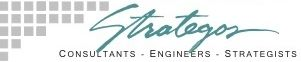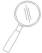The Economic Lot Size Model

An Old Theory Still Has Value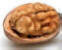In A Nutshell

Summary of the Economic Lot Size Model

Economic Lot Size (ELS) was first developed about 1913. It balances the costs of inventory against the costs of setup over a range of batch quantities. In this model, the Economic Lot Size (ELS) is where Total Cost is minimum.

Economic Lot Size (ELS) is dead according to some advocates of Lean Manufacturing and Theory of Constraints. They contend that every operation should manufacture what the downstream customer needs immediately in "batches" of one unit. (One Piece Flow)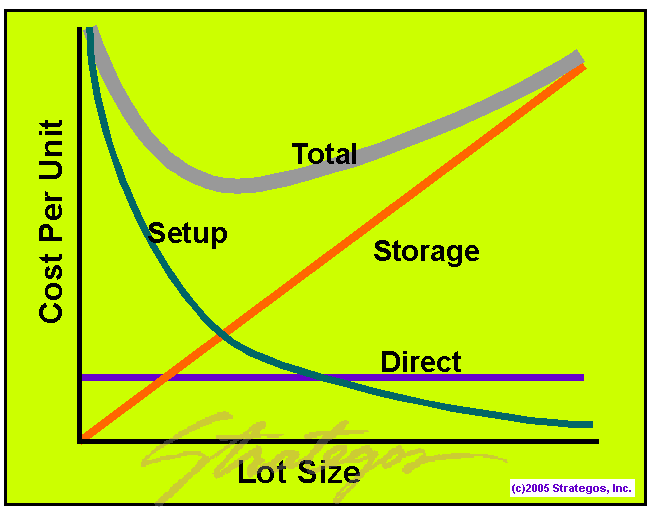This may be correct in an ideal world. Most factories are less than ideal. Where significant setup costs exist, batch quantity is still an issue and Economic Lot Size provides important insights for rational decisions.

The figure depicts a typical ELS model. This model calculates the total production cost per unit over a range of batches. The batch quantity having the lowest unit cost is the ideal or Economic Lot Size. This Total Cost typically forms a "U" as shown in the figure.

The model classifies total cost into three components: Setup Cost, Direct Cost and Carrying Cost (Storage Cost). This facilitates calculation and aids understanding.

Direct Cost

Direct costs are directly proportional to the amount produced. Materials and direct labor are the most common. Accounting systems usually capture these costs accurately and make them readily available. In the figure, direct cost per piece is a horizontal line for all batch quantities.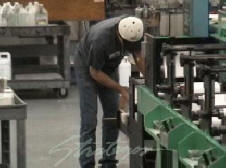Printing Press Setup

Setup (or make-ready) is critical in the printing industry.

Setup Cost

Setup costs include the labor and material to ready a machine for production. They may include the processing of work orders or a first-article inspection. We amortize these costs over the entire batch to derive the Setup Cost per piece. This cost is high when batches are small and rapidly decreases with increasing batch quantity.

Carrying Cost

Carrying or Storage cost is the average cost associated with storing an average production unit for the average time it will be in inventory. These costs are more difficult to calculate and we will not take up that procedure here. Storage costs are significant and often represent 20%-60% of inventory value on an annual basis.

Carrying Cost per piece (in the simplest case) varies directly with batch quantity. The larger the batch, the more units will be in inventory, on average.

There are problems with using ELS as the only determinate. The next page explains how to make rational batching decisions using ELS as one of several considerations.

■ ■ ■ ■ ■ ■ ■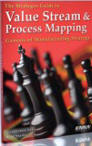The Strategos Guide To Value Stream and Process Mapping goes  beyond symbols and arrows. In over 163 pages it tells the reader how to do it and what to do with it.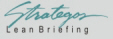The free newsletter of Lean strategy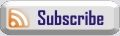Books & Videos
 Contact Webmaster SEP 2007